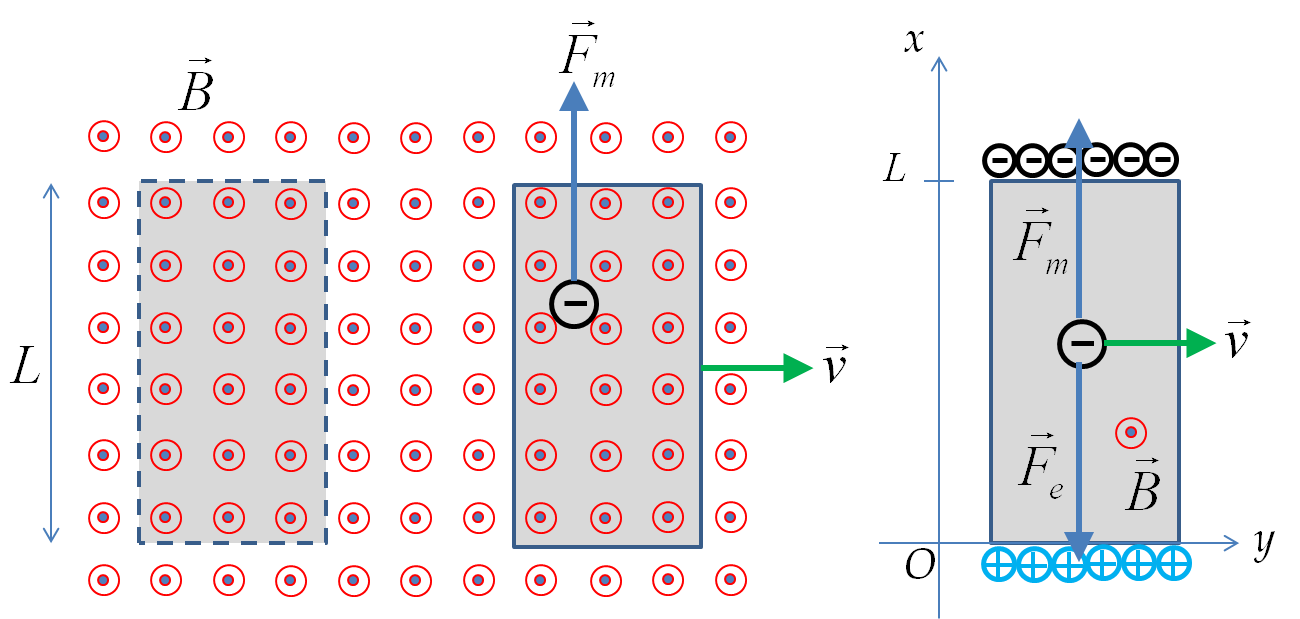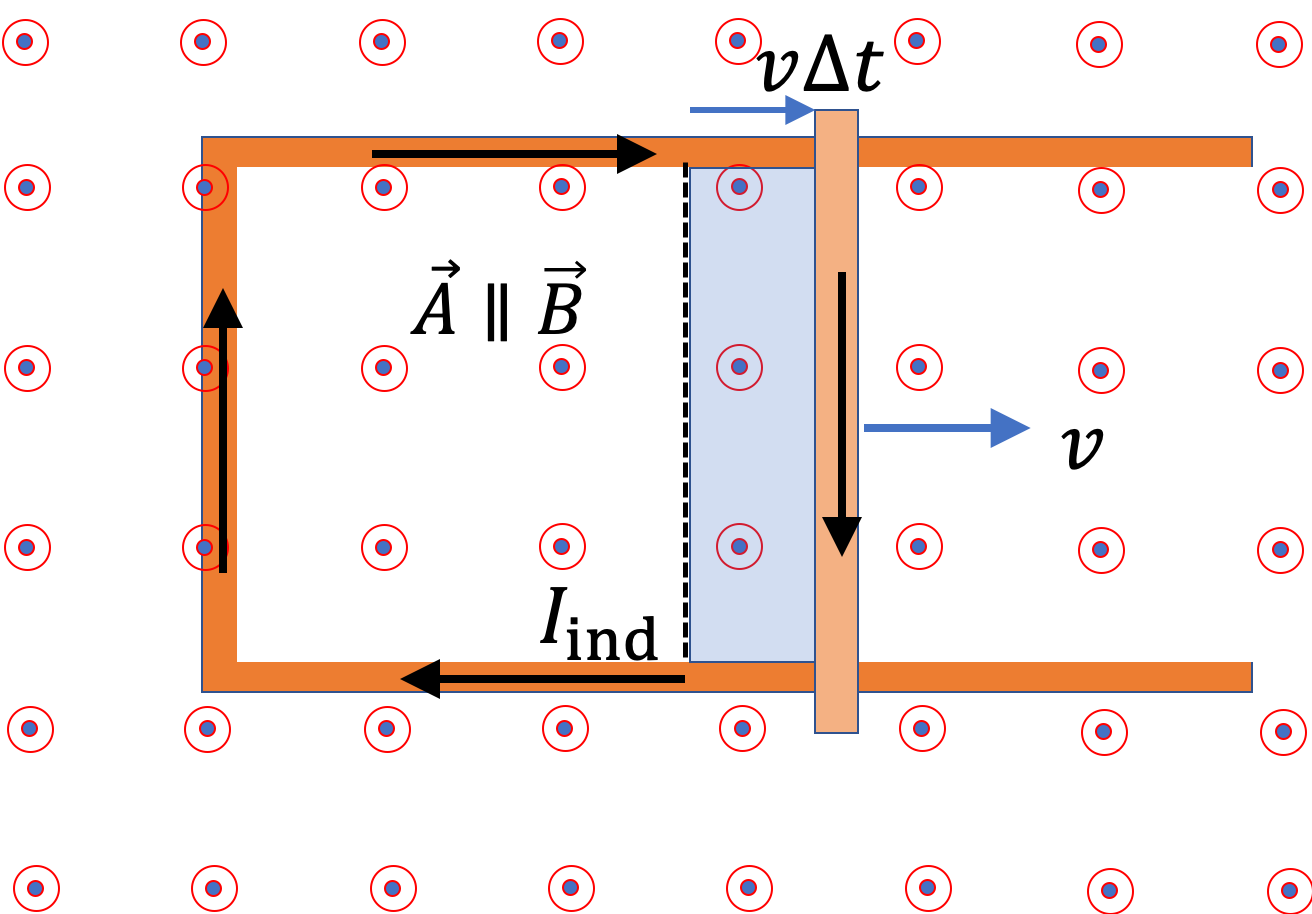## Section41.4Motional EMF

Faraday's flux rule states that a changing magnetic flux through an open surface induced an EMF in the closed loop around the surface. The induced EMF is equal to the rate at which magnetic flux through the surface chages.

\begin{equation*} \mathcal{E}_\text{ind} = -\dfrac{\Delta \Phi_B}{\Delta t}. \end{equation*}

The flux change can occur in three ways with each contributing to the induced EMF.

1. Changing magnetic field magnitude
2. Changing area of the surface
3. Changing orientation of the surface with respect to the magnetic field.

Both changing of area and orientation involve motion and hence the EMF induced as a result of motion are called motional EMF. In this section we will illustrate motional EMF in a moving bar.

### Subsection41.4.1Moving Conductor in a Magnetic Field

You may remember a useful rule that a conductor has same electric potential at all points. Turns out that this rule is true only for static conditions. When a conductor is moving in a magnetic field, Lorentz force on conduction electrons can polarize the metal. This leads to a potential difference in the conductor even in the absence of any voltage source! We will work out a formula for this voltage now.

Consider a neutral metallic bar moving with velocity $\vec v$ in a region of magnetic field $\vec B$ perpendicular to the velocity as shown in Figure 41.4.1. The conduction elctrons in the metal are free to move while positive nuclei are fixed in place. Therefore, magnetic force on conduction electrons will accelerate them.Figure 41.4.1. Magnetic force on conduction electrons accelerates them to one end of the moving rod. With accumulation of charges, electric field in the rod develops which opposes the magnetic force. For steady speed motion, the two forces balance and prevent further accumulation.

Since accelerating electrons cannot leave the bar, they will start to accumulate at one end, making that end negative relative to the other end of the bar, which becomes positive. Resulting polarization of the bar creates electric field $\vec E$ that opposes the magnetic force. An equilibrium is reached for a bar that is moving a constant speed. At equilibrium, we must have the following for every electron.

\begin{equation} -e v B = - e E\Longrightarrow E = v B.\label{eq-moving-bar-magnetic-force-balance}\tag{41.4.1} \end{equation}

Let $l$ be the length of the bar. Then, assuming $E$ to be constant throughout the moving bar, we can state that the two ends will have a potential difference given by the following.

\begin{equation} \phi_+ - \phi_- = E l = v B l.\label{eq-moving-bar-potential-difference}\tag{41.4.2} \end{equation}

This potential difference disappears very soon after the bar stops moving. Since, this phenomenon is purely dynamical, a better way for looking at this is through Faraday's law.

In terms of Faraday's law, we think of the moving bar as part of a fictitious circuit shown dashed in Figure 41.4.2. When bar is moving, the magnetic flux through the fictious loop is varying at rate equal to $Bvl\text{.}$ Therefore, we expect an EMF to be induced in the circuit of this amount.

\begin{equation} \left| \mathcal{E}_\text{ind}\right| = Bvl.\label{eq-motional-emf-from-force}\tag{41.4.3} \end{equation}

### Subsection41.4.2Moving Metal Bar on a U-Shaped Metal

Now, we examine what will happen if electrons in a moving conductor did not accumulate, but they coul continue their drift in some other conductor. To provide a continuous path to drifting electrons, we slide the bar on a U-shaped metal as shown in Figure 41.4.3.

Even though charges will not accumulate anywhere in the loop made by the U-shaped metal and moving cross bar, the motion of the cross bar will continuously accelerate charges, which will cause drift of electrons. The traditional current direction is shown in the opposite direction to the drift of electrons in Figure 41.4.3.Figure 41.4.3. Metal sliding bar in a magnetic field. There are two equivalent ways of explaining the generation of induced current in the closed conducting loop made by the bar and the U-shaped metal. In the Lorentz force picture, we look at the EMF by the magnetic force on mobile charges of the metal bar. In the flux rule picture, we look at the expanding area of the surface enclosed by the loop. The shaded part shows the amount the horizontal dimension of the loop expands in time $\Delta t\text{.}$

We can compute the amount of work is done per unit charge for moving the charge around the circuit once, i.e., the EMF induced in teh circuit, in the following two equivalent ways.

1. Using Lorentz Force

Since only the bar is moving, the electric field will be same as we worked out in (41.4.1), from which, we will find that the induced EMF has magnitude given by Eq. (41.4.3).

\begin{equation} \mathcal{E}_\text{ind} = vBl.\label{eq-motional-emf-from-force-reproduced}\tag{41.4.4} \end{equation}

Since we have a loop associated with this force, we can actullay, use Faraday flux rule to get this answer as we show next.

We can understand Eq. (41.4.4) from Faraday's flux rule in Eq. (41.3.1) or (41.3.3) if we think of the cross-bar making a closed loop with the U-shaped metal. Therefore, instead of interperting current induced by Lorentz force as in Eq. (41.4.3), we can actually, think of this emf being induced by changing area, i.e., $\Delta \Phi_{B,A}\text{.}$ During $t$ to $t+\Delta t\text{,}$ the bar moves a distance $v\Delta t$ and hence the area of the loop increases by

\begin{equation*} \Delta A = l v\Delta t, \end{equation*}

which is shown shaded in Figure 41.4.3. Therefore, induced EMF will be

\begin{align*} \mathcal{E}_\text{ind} \amp = - \dfrac{\Delta \Phi_{B,B}}{\Delta t} -\dfrac{\Delta \Phi_{B,A}}{\Delta t} - \dfrac{\Delta \Phi_{B,\theta}}{\Delta t}, \\ \amp = -\dfrac{\Delta \Phi_{B,A}}{\Delta t}, \\ \amp = - B \cos 0^\circ \dfrac{\Delta A}{\Delta t}, \\ \amp = - B l v, \end{align*}

which has the same magnitude as in Eq. (41.4.3), the motional EMF found by looking at force on the conducting electrons. The sign here tells us that induced current when bar is moving out, will be in the clockwise sense when viewed from the area vector direction, i.e., opposite of the area vector direction when we use the right-hand rule illustrated above.

Equivalent descriptions of moving bar induced EMF by Lorentz force and by flux rule clearly demonstrates that one should not just add EMF's from these two calculations since that would double count the EMF. A good practice is that you first examine all the EMF's that can be included by the flux rule, and then reexamine the circuit to find any Lorentz force-indeuced EMF that has not been accounted for.

### Subsection41.4.3Energy Dissipation by Induced Current

We have found that when the area of a conducting loop changes, an induced current flows in the loop. Say the loop has some resistance $R\text{,}$ the flowing current in the conductor will result in the heating of the conductor and energy will exit the loop at the following rate.

\begin{equation*} P = I_\text{ind}\,R^2. \end{equation*}

Where does this energy come from? Magnetic force due to velocity $v$ of the movement of the bar as a whole is along the wire, but magnetic force on the current in the bar is pointed opposite to the velocity of the bar. Thus, to maintain the velocity at $\vec v\text{,}$ an external force $\vec F$ must be pulling the bar with force that balances the magetic force on the bar of length $l\text{.}$

\begin{equation*} F = I_\text{ind}\,Bl. \end{equation*}

The rate at which this force does work is

\begin{equation*} \dfrac{W}{\Delta t} = F v = I_\text{ind}\,Bl v. \end{equation*}

The EMF in the circuit is $Blv\text{.}$ Therefore,

\begin{equation*} \dfrac{W}{\Delta t} = I_\text{ind}\mathcal{E}_\text{ind}. \end{equation*}

Now, using Ohm's law we can write this as

\begin{equation*} \dfrac{W}{\Delta t} = I_\text{ind}\, R^2. \end{equation*}

We find that the energy for current in the current loop is provided by an external agent that is moving the bar.

A metal bar of mass 500 g slides outward at a constant speed of 1.5 cm/s over two parallel rails separated by a distance of 30 cm which are part of a U-shaped conductor. There is a uniform magnetic field of magnitude 2 T pointing out-of page over the entire area. The railings and the metal bar have an equivalent resistance of 150 $\Omega\text{.}$

(a) Determine the induced current, both magnitude and direction.

(b) Find the direction of the induced current if the magnetic field is pointed into the page.

(c) Find the direction of the induced current if the magnetic field is pointed into the page and the bar moves inwards.

Hint

Use flux rule and Lenz's law.

(a) $6\times 10^{-5} \ \text{A}\text{,}$ (b) counterclockwise, (c) clockwise.

Solution 1 (a)

(a) The magnitude of induced current is equal to induced EMF divided by equivalent resistance. $I_{\text{ind}} = \dfrac{\left|\Delta \Phi/\Delta t \right|}{R_{\text{eq}}} = \dfrac{2\:\text{T}\times0.3\:\text{m}\times 0.015\:\text{m/s}}{150\:\Omega} = 6\times 10^{-5}\:\text{A}.$ The direction of the induced current will be such that its magnetic field will tend to oppose the change in magnetic flux through the loop. Hence, we need in-the-page direction for the induced magnetic field. Hence, induce current will be clock.wise.

Solution 2 (b)

(b) The induced EMF will be in the counterclockwise direction for a magnetic field pointed into-the-page.

Solution 3 (c)

(c) If the magnetic field was pointed into-the-page and the metal bar moved inward, then the induced magnetic field must be also into-the-page in order to oppose the change in the magnetic flux. Hence, the induced current will be in the clockwise direction as seen from above.